Get familiar with gradients in this math quiz.

# Straight Line 1

This Math quiz is called 'Straight Line 1' and it has been written by teachers to help you if you are studying the subject at high school. Playing educational quizzes is a user-friendly way to learn if you are in the 9th or 10th grade - aged 14 to 16.

It costs only \$12.50 per month to play this quiz and over 3,500 others that help you with your school work. You can subscribe on the page at Join Us

In the Linear Equations quizzes you practiced solving equations using algebra. This high school Math quiz should help you understand the link between the algebra and the geometry of the straight line.

When a straight line is plotted on an x-y grid there are two key features that we can obtain, which will allow us to write down the equation of the straight line. This equation is the rule that connects the x- and the y-values.

The first feature is the gradient, which is a measure of how steep the line is. Recall that the gradient is ‘change in height over change in distance’. To calculate the gradient, plot two points on the line that pass directly through the crosshairs of the grid.

Form a right triangle, then count how many units up or down – this is change in height – and how many units left or right, which is change in distance. Then you need to decide if it is positive (goes up from left to right) or negative (goes down from left to right). Gradient is denoted using the letter m.

The other feature is the y-axis intercept. This is the number on the y-axis (vertical) that the line passes through, and is represented using the letter c.

You obtain the equation of the line by substituting the values for m and c into the general equation of the straight line, y = mx + c.

The equation of horizontal and vertical lines often catches people out. On a horizontal line, the y-value of any point is the same. This means the equation is of the form y = …. The same logic applies to a vertical line – the x-value of any point on the line will be the same, so its equation is x = ….

Question 1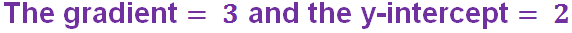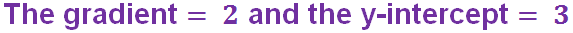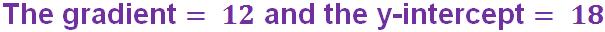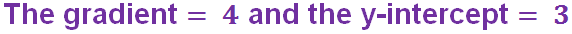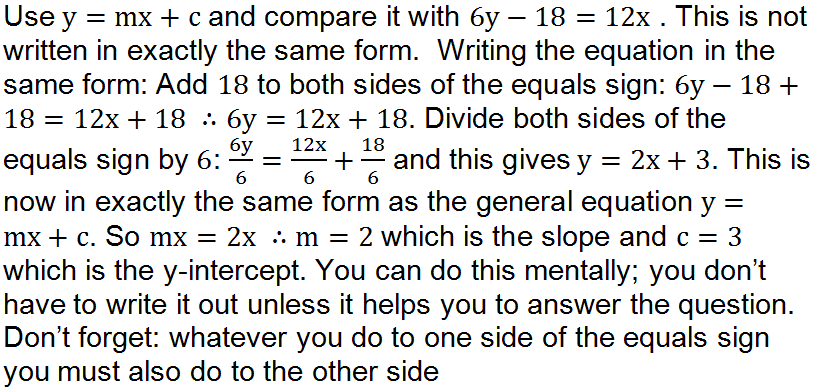Question 2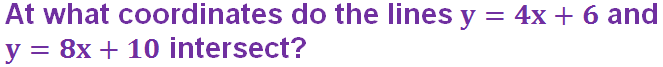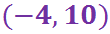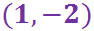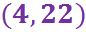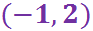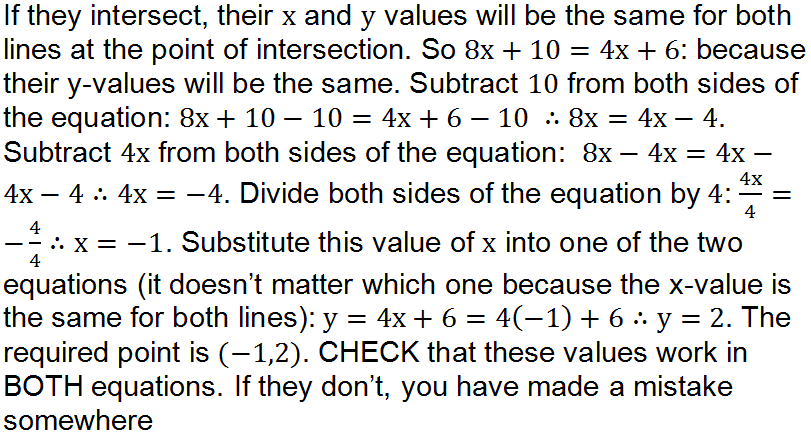Question 3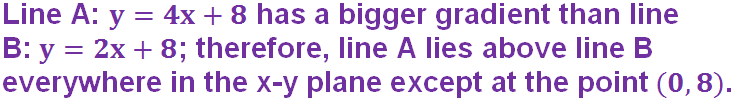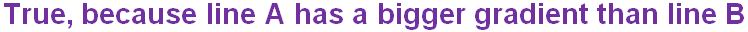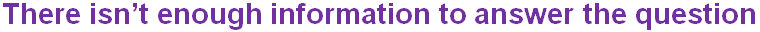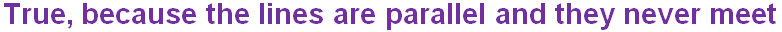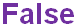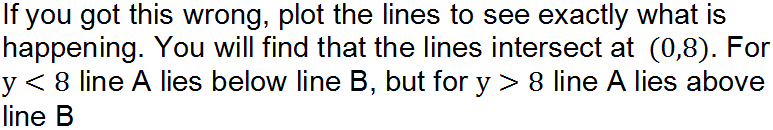Question 4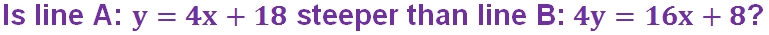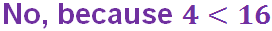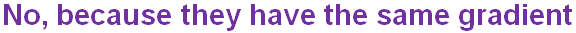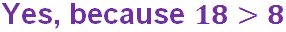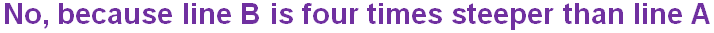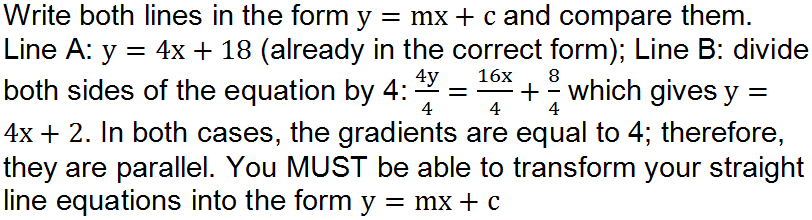Question 5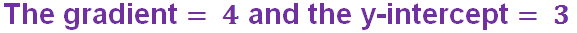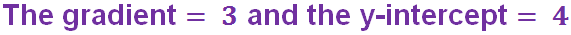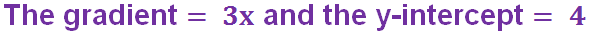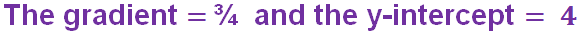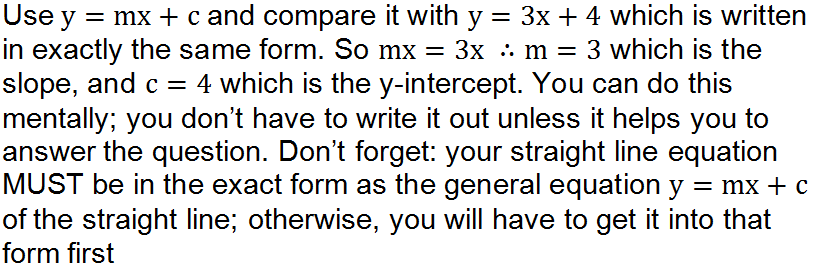Question 6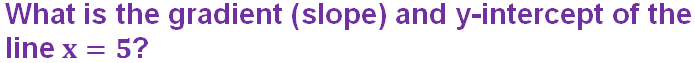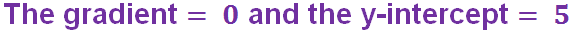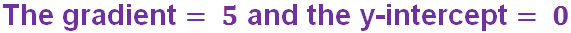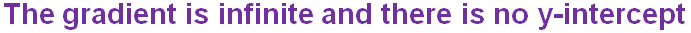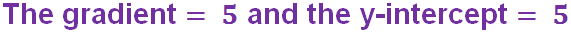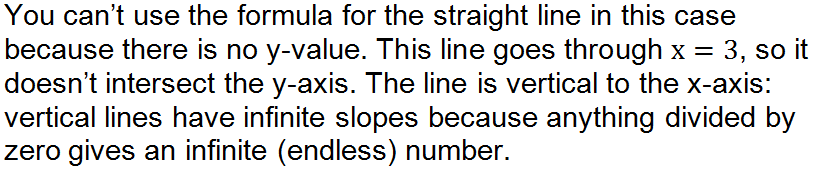Question 7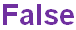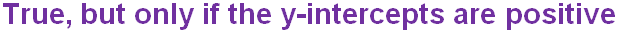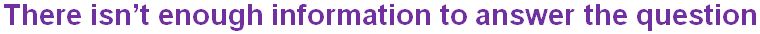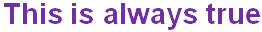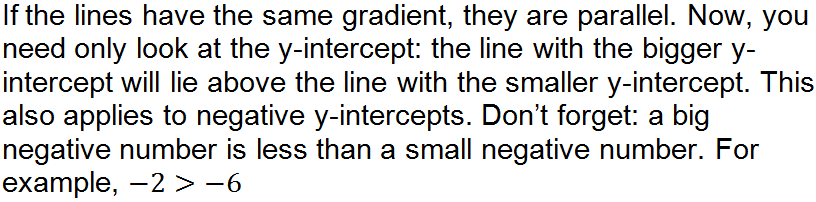Question 8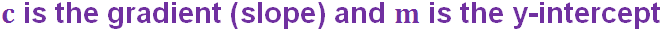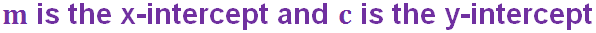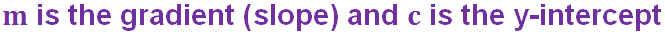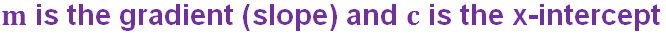Question 9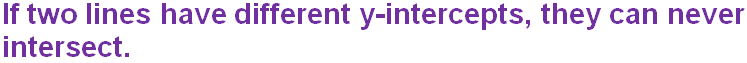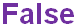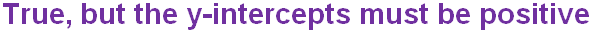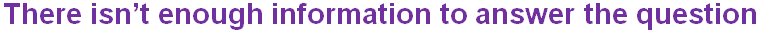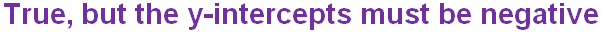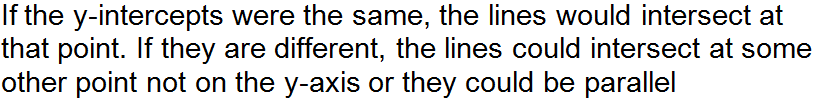Question 10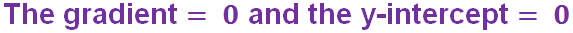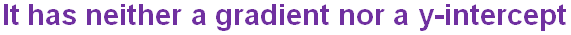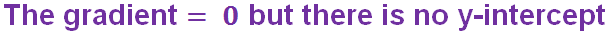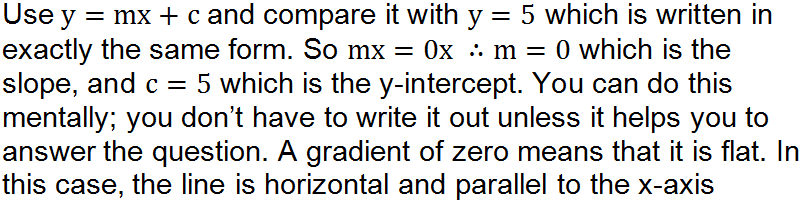Author:  Frank Evans